# Samacheer Kalvi 6th Maths Solutions Term 2 Chapter 2 Measurements Ex 2.2

## Tamilnadu Samacheer Kalvi 6th Maths Solutions Term 2 Chapter 2 Measurements Ex 2.2

Samacheer Kalvi 6th Maths Book Solutions Term 2 Question 1.
Say the time in two ways: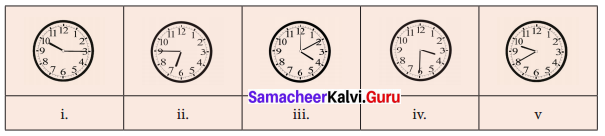Solution:
(i) 10 : 15 hours; quarter past 10; 45 minutes to 11
(ii) 6 : 45 hours; quarter to 7; 45 minutes past 6
(iii) 4 : 10 hours; 10 minutes past 4; 50 minutes to 5
(iv) 3 : 30 hours; half-past 3; 30 minutes to 4
(v) 9 : 40 hours; 20 minutes to 10; 40 minutes past 9.

6th Maths Guide Term 2 Question 2.
Match the following:
(i) 9.55 – (a) 20 minutes past 2
(ii) 11.50 – (b) quarter past 4
(iii) 4.15 – (c) quarter to 8
(iv) 7.45 – (d) 5 minutes to 10
(v) 2.20 – (e) 10 minutes to 1?
Solution:
(i) 9.55 – (d) 5 minutes to 10
(ii) 11.50 – (e) 10 minutes to 12
(iii) 4.15 – (b) quarter past 4
(iv) 7.45 – (c) quarter to 8
(v) 2.20 – (a) 20 minutes past 2

Samacheer Kalvi 6th Maths Term 2 Question 3.
Convert the following :
(i) 20 minutes into seconds
(ii) 5 hours 35 minutes 40 seconds into seconds
(iii) 3$$\frac { 1 }{ 2 }$$ hours into minutes
(iv) 580 minutes into hours
(v) 25200 seconds into hours
Solution:
(i) 20 minutes into seconds
20 minutes = 20 × 60 seconds = 1200 seconds
(ii) 5 hours 35 minutes 40 seconds into seconds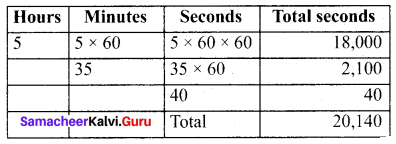∴ 5 hours 35 minutes 40 seconds = 20,140 seconds
(iii) 3$$\frac { 1 }{ 2 }$$ hours into minutes
3$$\frac { 1 }{ 2 }$$ hours = 3 hours 30 minutes
= 3 × 60 minutes + 30 minutes
= 180 minutes + 30 minutes
= 210 minutes
∴ 3$$\frac { 1 }{ 2 }$$ hours = 210 minutes
(iv) 5580 minutes into hours
580 minutes = $$\frac{580}{60}$$ hours = 9 hours 40 minutes
∴ 580 minutes = 9 hours 40 minutes
(v) 25200 seconds into hours
25200 seconds = $$\frac{25200}{60}$$ minutes = 420 minutes = $$\frac{420}{60}$$ hours = 7 hours
∴ 25200 seconds = 7 hours

Samacheer Kalvi 6th Maths Book Solutions Question 4.
The duration of electricity consumed by the farmer for his pumpset on Monday and Tuesday was 7 hours 20 minutes 35 seconds and 3 hours 44 minutes 50 seconds respectively. Find the total duration of consumption of electricity.
Solution:
Total duration of electricity consumed on both the days
= 7 hours 20 min 35 sec + 3 hours 44 min 50 sec
= (7 + 3) hours (20 + 44) min (35 + 50) sec
= 10 hours 64 min 85 seconds
= 11 hours 5 min 25 seconds

6th Standard Maths Exercise 2.2 Question 5.
Subtract 10 hrs 20 min 35 sec from 12 hrs 18 min 40 sec.
Solution: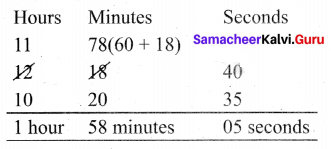1 hour 58 minutes 05 seconds

Samacheer Kalvi Term 2 Question 6.
Change the following into 12 hour format:
(i) 02 : 00 hours
(ii) 08 : 45 hours
(iii) 21 : 10 hours
(iv) 11 : 20 hours
(v) 00 : 00 hours
Solution: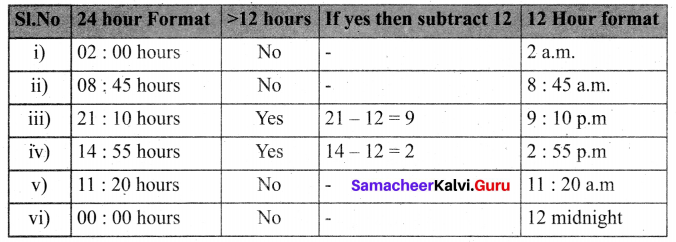Samacheer Kalvi 6th Maths Question 7.
Change the following into 24 hour format.
(i) 3.15 a.m.
(ii) 12.35 p.m.
(iii) 12.00 noon
(iv) 12.00 midnight.
Solution: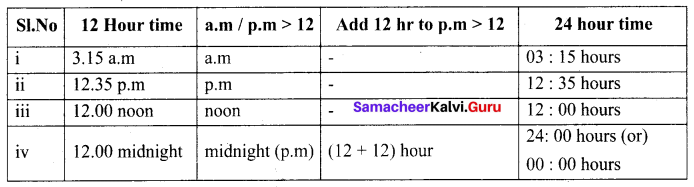6th Maths Term 2 Question 8.
Calculate the duration of time.
(i) from 5.30 a.m to 12.40 p.m
(ii) from 1.30 p.m to 10.25 p.m
(iii) from 20:00 hours to 4:00 hours
(iv) from 17:00 hours to 5 :15 hours
Solution:
(i) from 5.30 a.m. to 12 .40 p.m.
Duration of time from 5.30 a.m. to noon = 12 : 00 – 5 : 30 = 6 : 30 i.e 6 hours 30 minutes
From noon to 12.40 p.m the duration = 00 hours 40 minutes
Total duration = 6 hours 30 minutes + 00 hours 40 minutes
= 6 hours 70 minutes
= 6 hours + (60 + 10) minutes
= 6 hours + 1 hr 10 minutes
= 7 hours 10 minutes
∴ Duration of time from 5.30 am to 12.40 pm = 7 hours 10 minutes
(ii) from 1.30 pm to 10.25 p.m.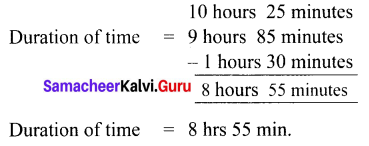(iii) from 20 : 00 hrs to 4 : 00 hrs.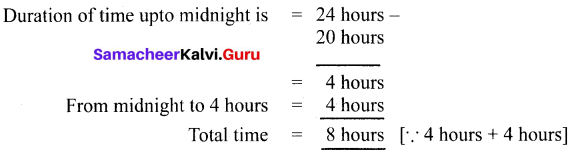(iv) from 17 : 00 hours to 5 : 15 hours.Term 2 Samacheer Kalvi Question 9.
The departure and arrival timing of the Vaigai Superfast Express (No. 12635) from Chennai Egmore to Madurai Junction are given. Read the details and answer the following.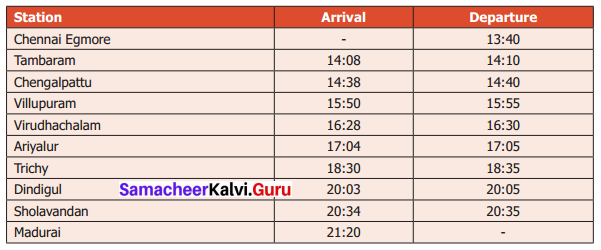(i) At what time does the Vaigai Express start from Chennai and arrive at Madurai?
(ii) How many halts are between there Chennai and Madurai?
(iii) How long does the train halt at the Villupuram junction?
(iv) At what time does the train come to Sholavandan?
(v) Find the journey time from Chennai Egmore to Madurai?
Solution:
(i) It starts from Chennai at 13 : 40 hrs and arrive at Madurai at 21 : 20 hrs.
(ii) There are 8 halts.
(iii) Departure from Villupuram = 15 hours 55 minutes
Arrival at Villupuram = 15 hours 50 minutes
The train halt at Villupuram for = 05 minutes
(iv) At 20 : 34 hours the train come to Sholavandan
(v) Arrival time at Madurai = 20 hours 80 (20 + 60) minutes
Departure time from Chennai Egmore = 13 hours 40 minutes
Journey Time = 07 hours 40 minutes

Samacheer Kalvi 6th Standard Maths Question 10.
Manickam joined a chess class on 20.02.2017 and due to exam, he left practice after 20 days. Again he continued practice from 10.07.2017 to 31.03.2018. Calculate how many days did he practice?
Solution:
From the date of joining = 20 days From 10.07.2017 to 31.03.2018
July – 22
Aug – 31
Sep – 30
Oct – 31
Nov – 30
Dec – 31
Jan – 31
Feb – 28
Mar – 31
Total – 265
Total no of practice days = 265 + 20 = 285 days

6th Term 2 Maths Guide Question 11.
A clock gains 3 minutes every hour. If the clock is set correctly at 5 a.m, find the time shown by the clock at 7 p.m?
Solution:
5 a.m. = 5.00 hours
7 p.m = 19.00 hours
Difference = 19.00 – 5.00 = 14.00 hours
Time gain = 14 × 3 = 42 minutes
Time shown by the clock = 7.42 p.m

Class 6 Maths Chapter 2 Exercise 2.2 Solutions Question 12.
Find the number of days between the republic day and kalvi valarchi day in 2020.
Solution:
2020 is a leap year republic day – 26.01.2020
Kalvi valarchi day – 15.07.2020
Jan – 5
Feb – 29
Mar – 31
April – 30
May – 31
June – 30
July – 14
Total – 170 days

Question 13.
If 11th of January 2018 is Thursday, what is the day on 20th July of the same year?
Solution:
January – 21 Days (31 – 10)
February – 28 Days
March – 31 Days
April – 30 Days
May – 31 Days
June – 30 Days
July – 19 Days
Total – 190 Days
190 days ÷ 7
190 days = 27 weeks + 1 day
Required day is the first day after Thursday.
∴ 20th of July is Friday.

Question 14.
(i) Convert 480 days into years
(ii) Convert 38 months into years
Solution:
(i) 480 days = $$\frac{480}{365}$$
= 1 year 115 days
= 1 year 3 months 25 days

(ii) 38 months = $$\frac{38}{12}$$
= 3 years 2 months

Question 15.
Calculate your age as on 01.06.2018
Solution: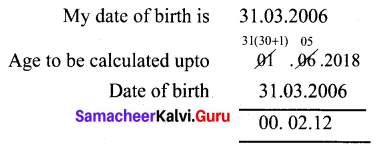Age is 12 years 2 months

Objective Type Questions

Question 16.
2 days = …….. hours
(i) 38
(ii) 48
(iii) 28
(iv) 40
Solution:
(ii) 48

Question 17.
3 weeks = _____ days.
(a) 21
(b) 7
(c) 14
(d) 28
Solution:
(a) 21

Question 18.
Number of ordinary years between two consecutive leap years is
(i) 4 years
(ii) 2 years
(iii) 1 year
(iv) 3 years
Solution:
(iv) 3 years

Question 19.
What time will it 5 hours after 22 : 35 hours?
(a) 2 : 30 hrs
(b) 3 : 35 hrs
(c) 4 : 35 hrs
(d) 5 : 35 hrs
Solution:
(b) 3 : 35 hrs

Question 20.
2 $$\frac{1}{2}$$ years is equal to months.
(i) 25
(ii) 30
(iii) 24
(iv) 5
Solution:
(ii) 30